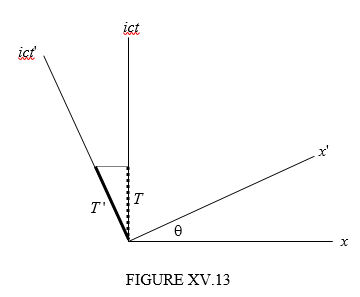$$\require{cancel}$$

# 15.10: Time Dilation

$$\newcommand{\vecs}{\overset { \rightharpoonup} {\mathbf{#1}} }$$ $$\newcommand{\vecd}{\overset{-\!-\!\rightharpoonup}{\vphantom{a}\smash {#1}}}$$$$\newcommand{\id}{\mathrm{id}}$$ $$\newcommand{\Span}{\mathrm{span}}$$ $$\newcommand{\kernel}{\mathrm{null}\,}$$ $$\newcommand{\range}{\mathrm{range}\,}$$ $$\newcommand{\RealPart}{\mathrm{Re}}$$ $$\newcommand{\ImaginaryPart}{\mathrm{Im}}$$ $$\newcommand{\Argument}{\mathrm{Arg}}$$ $$\newcommand{\norm}{\| #1 \|}$$ $$\newcommand{\inner}{\langle #1, #2 \rangle}$$ $$\newcommand{\Span}{\mathrm{span}}$$ $$\newcommand{\id}{\mathrm{id}}$$ $$\newcommand{\Span}{\mathrm{span}}$$ $$\newcommand{\kernel}{\mathrm{null}\,}$$ $$\newcommand{\range}{\mathrm{range}\,}$$ $$\newcommand{\RealPart}{\mathrm{Re}}$$ $$\newcommand{\ImaginaryPart}{\mathrm{Im}}$$ $$\newcommand{\Argument}{\mathrm{Arg}}$$ $$\newcommand{\norm}{\| #1 \|}$$ $$\newcommand{\inner}{\langle #1, #2 \rangle}$$ $$\newcommand{\Span}{\mathrm{span}}$$

We imagine the same railway train $$\sum'$$ and the same railway station $$\sum$$ as in the previous section except that, rather than measuring a length referred to the two reference frames, we measure the time interval between two events. We’ll suppose that a passenger in the railway train $$\sum'$$ claps his hands twice. These are two events which, when referred to the reference frame $$\sum'$$, take place at the same place when referred to this reference frame. Let the instants of time when the two events occur, referred to $$\sum'$$, be $$t'_{1}$$ and $$t'_{2}$$. The time interval $$T'$$ is defined as $$t'_{2}-t'_{1}$$. But the Lorentz transformation is

$t=\gamma(t'+\frac{\nu x'}{c^{2}}) \nonumber$

and so the time interval when referred to $$\sum$$ is

$T=\gamma T'. \label{15.10.1}$

This is the dilation of time. The situation is illustrated by a Minkowski diagram in Figure XV.13. While it is clear from the figure that $$T=T'\cos\theta$$ and therefore that $$T=\gamma T'$$ it is not so clear from the figure that this means that $$T$$ is greater than $$T'$$ – because $$\cos\theta > 1$$ and $$\theta$$ is imaginary.Thus, let us suppose that a passenger on the train holds a 1-metre measuring rod (its length in the direction of motion of the train) and he claps his hands at an interval of one second apart. Let’s suppose that the train is moving at 98% of the speed of light ($$\gamma$$ = 5.025). In that case the stationmaster thinks that the length of the rod is only 19.9 cm and that the time interval between the claps is 5.025 seconds.

I deliberately did not word that last sentence very well. It is not a matter of what the stationmaster or anyone else “thinks” or “asserts”. It is not a matter that the stationmaster is somehow deceived into erroneously believing that the rod is 19.9 cm long and the claps 5.025 seconds apart, whereas they are “really” 1 metre long and 1 second apart. It is a matter of how length and time are defined (by subtracting two space coordinates determined at the same time, or two time coordinates at the same place) and how space-time coordinates are defined by means of the Lorentz transformations. The length is 19.9 cm, and the time interval is 5.025 seconds when referred to the frame $$\sum$$. It is true that the proper length and the proper time interval are the length and the time interval referred to a frame in which the rod and the clapper are at rest. In that sense one could loosely say that they are “really” 1 metre long and 1 second apart. But the Lorentz contraction and the time dilation are not determined by what the stationmaster or anyone else “thinks”.

Another way of looking at it is this. The interval $$s$$ between two events is clearly independent of the orientation any reference frames, and is the same when referred to two reference frames that may be inclined to each other. But the components of the vector joining two events, or their projections on to the time axis or a space axis are not at all expected to be equal.

By the way, in Section 15.3 I urged you to write a computer or calculator programme for the instant conversion between the several factors commonly encountered in relativity. I still urge it. As soon as I typed that the train was travelling at 98% of the speed of light, I was instantly able to generate $$\gamma$$. You need to be able to do that, too.

This page titled 15.10: Time Dilation is shared under a CC BY-NC 4.0 license and was authored, remixed, and/or curated by Jeremy Tatum via source content that was edited to the style and standards of the LibreTexts platform; a detailed edit history is available upon request.